JEE  >  Differentiation for Physics

# Differentiation for Physics - Notes | Study Physics For JEE - JEE

 1 Crore+ students have signed up on EduRev. Have you?

Function: If the value of a quantity y (say) depends on the value of another quantity x, then y is the function of x i.e. y = f(x).
The quantity y is called dependent variable and the quantity x is called independent variable. For example, y = 2x2 + 4x + 7 is a function of x
(i) When x = 1, y = 2(1)2 + 4x1+7 = 13
(ii) When x = 2, y = 2(2)2 +4x2+7 = 23
As the value of y depends on the value of x, y is the function of x.

Differential coefficient or derivative of a function
Let y = f(x) …. (1)
That is, the value of y depends upon the value of x.
Let ∆x be a small increment in x, so that ∆y is the corresponding small increment in y, then
y + ∆y = f(x+∆x) …. (2)
Subtract (1) from (2), we get ∆y = f(x+∆x) − f(x)
Divide both sides by ∆x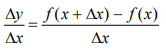Where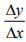is called average rate of change of y w.r.t. x.
Let us ∆x be as small as possible i.e. ∆x→0 (read as delta x tends to zero)
Then differential coefficient or derivative of y w.r.t. x is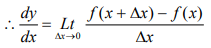Theorems of Differentiations

1. If y = C, when C is constant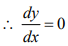2. If y = xn, where n is an integer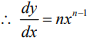3. If y = Cu, where u is the function of x and C is constant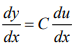4. If y = u ± v ± ω ± ……, where u, v and ω are the function of x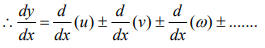5. If y = u v, where u and v are the function of x, then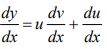6. If y =v/u , where u and v are the function of x, then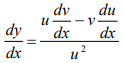7. If y = un, where u is the function of x then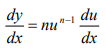Differential coefficients of Trigonometric Functions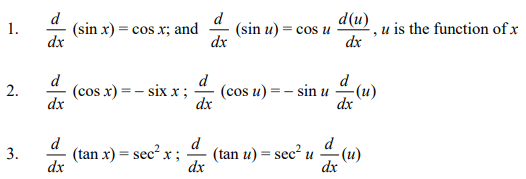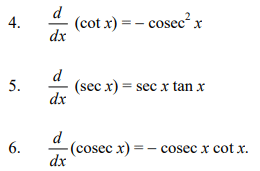Example: Differentiate the following w.r.t. x.

(i) sin 2 x
(ii) x sin x

(i) Let y = sin 2x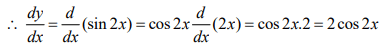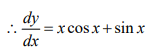(ii) Let y = x sin x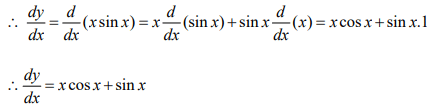Differential Coefficients of Logarithmic and Exponential Functions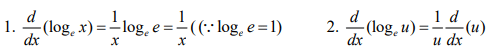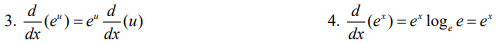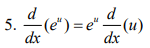Example: Differentiate the follow w.r.t .x.

(i) 2 (logx)2
(ii) log(ax + b)

(i) Let y = (loge x)2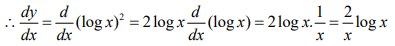(ii) Let y  = log(ax + b)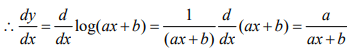Example: If S = 2t3 - 3t2 + 2,find the position, velocity and acceleration of a particle at the end of 2s. S is measured in metre and t in second

S = 2t3 - 3t2 + 2

When t = 2s, S = 2 × 8 – 3 × 4 + 2 = 6 m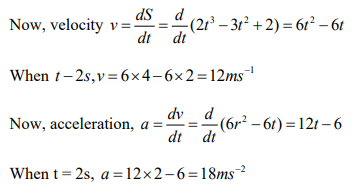The document Differentiation for Physics - Notes | Study Physics For JEE - JEE is a part of the JEE Course Physics For JEE.
All you need of JEE at this link: JEE

## Physics For JEE

257 videos|633 docs|256 tests
 Use Code STAYHOME200 and get INR 200 additional OFF

## Physics For JEE

257 videos|633 docs|256 tests

Track your progress, build streaks, highlight & save important lessons and more!

,

,

,

,

,

,

,

,

,

,

,

,

,

,

,

,

,

,

,

,

,

;